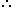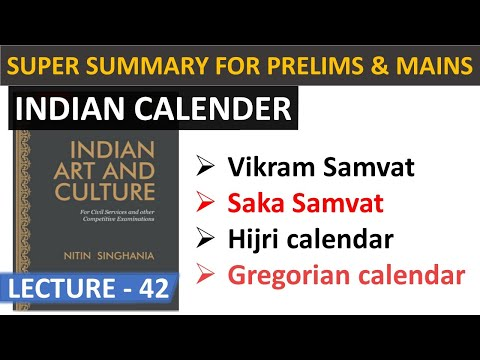Courses

# Test: Calendars- 2

## 10 Questions MCQ Test Quantitative Aptitude for Banking Preparation | Test: Calendars- 2

Description
This mock test of Test: Calendars- 2 for Quant helps you for every Quant entrance exam. This contains 10 Multiple Choice Questions for Quant Test: Calendars- 2 (mcq) to study with solutions a complete question bank. The solved questions answers in this Test: Calendars- 2 quiz give you a good mix of easy questions and tough questions. Quant students definitely take this Test: Calendars- 2 exercise for a better result in the exam. You can find other Test: Calendars- 2 extra questions, long questions & short questions for Quant on EduRev as well by searching above.
QUESTION: 1

### What was the day of the Week on 17th June 1998?

Solution:

17th June, 1998 = (1997 years + Period from 1.1.1998 to 17.6.1998)
Odd days in 1600 years = 0
Odd days in 300 years = (5 x 3) ≡ 1
97 years has 24 leap years + 73 ordinary years.
Number of odd days in 97 years ( 24 x 2 + 73) = 121 = 2 odd days.
Jan.         Feb.       March       April         May         June
(31     +     28     +     31     +     30     +     31     +     17) = 168 days
Therefore 168 days = 24 weeks = 0 odd day.
Total number of odd days = (0 + 1 + 2 + 0) = 3.
Given day is Wednesday.

QUESTION: 2

### On what dates of April 2001 did Wednesday fall?

Solution:

We shall find the day on 1st April, 2001.
1st April, 2001 = (2000 years + Period from 1.1.2001 to 1.4.2001)
Odd days in 1600 years = 0
Odd days in 400 years = 0
Jan. Feb. March April
(31 + 28 + 31 + 1)     = 91 days ≡ 0 odd days.
Total number of odd days = (0 + 0 + 0) = 0
On 1st April, 2001 it was Sunday.
In April, 2001 Wednesday falls on 4th, 11th, 18th and 25th

QUESTION: 3

### 16th July 1776,the day of the week was?

Solution:

16th July, 1776 = (1775 years + Period from 1st Jan, 1776 to 16th July, 1776)
Counting of odd days :
1600 years have 0 odd day
100 years have 5 odd days
75 years = (18 leap years + 57 ordinary years)
= [(18 x 2) + (57 x 1)]
= 93 (13 weeks + 2 days)
= 2 odd days
1775 years have (0 + 5 + 2) odd days = 7 odd days = 0 odd day
Jan   Feb   Mar   Apr   May   Jun   Jul
31 + 29 + 31 + 30 + 31 + 30 + 16
= 198 days
= (28 weeks + 2 days)
Total number of odd days = (0 + 2) = 2
Required day was 'Tuesday'.

QUESTION: 4

How many days are there in x weeks x days

Solution:

x weeks x days = (7x + x) days
= 8x days.

QUESTION: 5

What will be the day of the week 15th August 2010?

Solution:

15th August, 2010 = (2009 years + Period 1.1.2010 to 15.8.2010)
Odd days in 1600 years = 0
Odd days in 400 years = 0
9 years = (2 leap years + 7 ordinary years) = (2 x 2 + 7 x 1) = 11 odd days ≡ 4 odd days.
Jan.     Feb.   March   April     Mayb   June     July       Aug.
(31   +   28   +   31   +   30   +   31   +   30   +   31   +   15) = 227 days
∴ 227 days = (32 weeks + 3 days) ≡ 3 odd days.
Total number of odd days = (0 + 0 + 4 + 3) = 7 ≡ 0 odd days.
Given day is Sunday

QUESTION: 6

It was Sunday on Jan 1, 2006. What was the day of the Week Jan 1, 2010

Solution:

On 31st December, 2005 it was Saturday.
Number of odd days from the year 2006 to the year 2009 = (1 + 1 + 2 + 1) = 5 days.
∴ On 31st December 2009, it was Thursday.
Thus, on 1st Jan, 2010 it is Friday.

QUESTION: 7

Today is Monday. After 61 days, it will be:

Solution:

Each day of the week is repeated after 7 days.

So, after 63 days, it will be Monday.After 61 days, it will be Saturday.

QUESTION: 8

The last day of a Century cannot be

Solution:

100 years contain 5 odd days.
∴ Last day of 1st century is Friday.
200 years contain (5 x 2) ≡ 3 odd days.
∴ Last day of 2nd century is Wednesday.
300 years contain (5 x 3) = 15 ≡ 1 odd day.
∴ Last day of 3rd century is Monday.
400 years contain 0 odd day.
∴ Last day of 4th century is Sunday.
This cycle is repeated.
∴ Last day of a century cannot be Tuesday or Thursday or Saturday.

QUESTION: 9

On 8th Feb,2005 it was Tuesday.What was the day of the Week on 8th Feb,2004?

Solution:

The year 2004 is a leap year. It has 2 odd days.
∴ The day on 8th Feb, 2004 is 2 days before the day on 8th Feb, 2005.
Hence, this day is Sunday.

QUESTION: 10

What was the day of the week on 28th May 2006?

Solution:

28 May, 2006 = (2005 years + Period from 1.1.2006 to 28.5.2006)
Odd days in 1600 years = 0
Odd days in 400 years = 0
5 years = (4 ordinary years + 1 leap year) = (4 x 1 + 1 x 2) ≡ 6 odd days
Jan.         Feb.       March       April         May
(31     +     28     +     31     +     30     +     28 ) = 148 days
∴ 148 days = (21 weeks + 1 day) ≡ 1 odd day.
Total number of odd days = (0 + 0 + 6 + 1) = 7 ≡ 0 odd day.
Given day is Sunday.

Track your progress, build streaks, highlight & save important lessons and more!

### Similar Content### Related tests